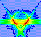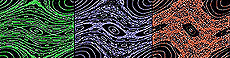Standard Map Main Page Previous | ContinuePhase Space By now, you have learned about one-dimensional maps, and about two-dimensional maps, both uncoupled and coupled. So far, however, we haven't said much about what the maps actually represent. That is, what do the mathematical transformations of the map tell us about the physical world?Standard Map Main Page Previous | ContinuePhase Space In the example of the two-dimensional map, for example, it was implied that the variables x and y that were transformed by the map might represent the horizontal and vertical positions of a point in space.Standard Map Main Page Previous | ContinuePhase Space That being so, one might expect that the interation of the map, which causes x and y to change in value, is representative of some physical process in which the horizontal and vertical locations of an object are changed repeatedly in time. The change in location of the object in time is mirrored by the change in the mathematical values under iteration of the map. ContinueStandard Map Main Page Previous | ContinuePhase Space Each iteration of the map would represent the change in the physical location of the object over a set time interval. That is, the time elapsed between each iteration of the map is always the same. For example, if the first iteration of the map represents the change in location after exactly one second, then the next iteration must represent the change after another one second, and so on.Standard Map Main Page Previous | ContinuePhase Space But although physicists usually use maps to represent physical processes in time, the two variables in two-dimensional maps don't usually represent the horizontal and vertical positions of the particles.Standard Map Main Page Previous | ContinuePhase Space Instead, the two-dimensional maps that interest physicists are the ones in which one variable of the map represents a position, but the other variable of the map represents a speed associated with the position.Standard Map Main Page Previous | ContinuePhase Space In other words, if one variable of two-dimensional map (x, for example) represents horizontal position, the other variable (y, for example) does not represent an actual vertical position, but instead it represents the horizontal speed of the particle at the same moment that the position is measured.Standard Map Main Page Previous | ContinuePhase Space Another example might be where one variable of the two-dimensional map represents the angular position of an object which is moving around in a circle. In this case, the other variable of the two-dimensional map would represent the angular speed, that is, the speed at which the object is moving around the circle.Standard Map Main Page Previous | ContinuePhase Space Like any two variables, the position and its associated speed can be plotted on a two-dimensional graph. But in this case, the two-dimensional graph does not represent a real two-dimensional area, since only one variable is a real position. ContinueStandard Map Main Page Previous | ContinuePhase Space The two-dimensional space formed by a real position and its associated speed is an abstract space that is called phase space by physicists. A phase space is any abstract space formed by a position (either linear or angular) and its associated speed. ContinueStandard Map Main Page Previous | ContinuePhase Space Phase space maps are the maps that physicists use to study the behavior of physical systems, including chaotic systems. The Standard Map, for example, is a phase space map. This means that in any graphical representation of the map, the plot does not actually represent what you would see with your eyes, since only one variable is a real position. Instead, the plots of phase space maps depict a general view of the motion, a view that is abstract, yet very powerful as tool for studying motion. Continue